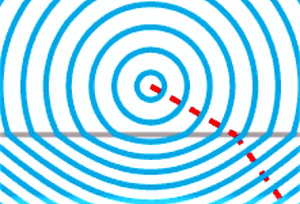Physics

# Snell’s law

The Snell law, is a formula that is used to know the relationship between the path taken by a ray of light when crossing the limit or the separation surface between two substances in contact and the refractive index of each of them. This law was discovered in 1621 by the Dutch astronomer and mathematician Willebrord Snell. The story of Snell's law was not published until Christiaan Huygens mentioned it in his treatise on light.Related topics

Reflection of light, refraction

## What's Snell's law?

Snell's law is a formula used to calculate the refraction angle of light when it passes through the separation surface between two different media.

It is important before explaining Snell’s law, to know also the definition of light refraction. This term refers to the change of direction that a wave can experience when it passes from one material medium to another. This change can only occur if the wave falls obliquely on the area of separation of the two media, and if they have different refractive indices.

So, Snell’s law consists of a formula that is particularly used to achieve the angle of refraction calculation of light when it passes the separation surface that exists between two media that have a different refraction index. The law then explains to us that the product of the refractive index by the sine of the angle of incidence is constant for any ray of light striking the surface of two media.

## History

Willebrord Snell van Royen was an astronomer and mathematician of Dutch origin who was born in 1580 in Leiden, and who died in 1626. He began to study law, but his main interest was mathematics. He also showed great interest in the study of physics and astronomy, and in 1615, he created a method to measure the Earth’s radius.

In 1621, he promulgated the law of refraction which was a simple formula for calculating the light’s angle of refraction when it passes through a surface that separates two media with different refractive indexes.

Apparently, it was first mentioned by Ibn Sahl in the 10th century. In 1601 the term was again taken into consideration by Thomas Harriot, but he did not publish it. The law was also described by René Descartes. Many scientists had analyzed that light rays changed direction when they came into contact with a denser surface, but Snell discovered the exact law in 1621.

## Snell’s law statement

The statement of Snell’s law tells us that the product of the index of refraction by the sine of the angle of incidence is constant for any ray of light striking the separating surface of two media. Although this law was created in order to explain the refraction phenomena of light, it can also be applied to all types of waves that are passing through a surface of separation between two media in which the velocity of propagation of the wave varies.

## Formula

The formula used to apply Snell’s law is as follows:

n1 . sen i= n2 . sen t , where:

i——> incident angle (it is the angle that forms the incident ray with the normal N).

t——> transmitted angle (it is the angle that forms the transmitted ray with the normal N).

n1—-> indicates the refractive index of the first medium.

n2—-> shows the refractive index of the second medium.

From the above equation it can be deduced that when n2<n1 there is an incidence angle, called the limit angle, from which there is no refraction——> Total reflection.

i_L->boundary angle : i_L=arc sen(n2/n1).

## Refraction

Refraction, in physics, refers to the change in the direction of a wave passing from one medium to another caused by its change in velocity. Light is refracted each time it travels at an angle towards a substance with a different refractive index, which is what we know as optical density. This change in direction is caused by a change in velocity.

## Applications of Snell’s law

Refraction allows us to have lenses, magnifiers, prisms and rainbows. Even our eyes depend on this curvature of light. Without refraction, we would not be able to focus light on our retina.

## Optical fiber

Fiber optics is a means of transmission that is mainly used in data networks. It consists of a very thin plastic or glass wire through which light pulses or data to be transmitted are sent. The light beam propagates through the fiber by means of a reflection angle that passes over the angle that is the limit of the total reflection. This light source used can be a laser or also an LED light.

The optical fibers are very used in telecommunications because they have the advantage of being able to take information through long distances to quite fast speeds.

## Examples

Some examples of Snell’s law are:

• When we observe a mirage, these are originated by extreme cases of refraction of light, and it is known with the name of total reflection.
• When we observe the mountains or trees reflected in the waters of rivers or lakes.
• In optical fibers.
Written by Gabriela Briceño V.